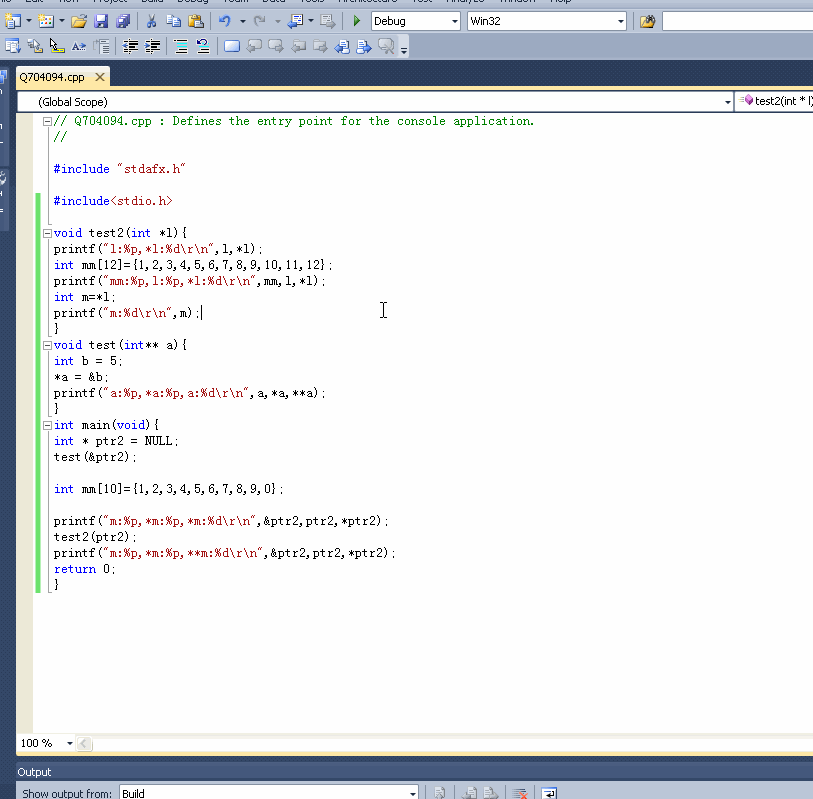C语言栈相关，指针操作以及局部变量函数返回的相关问题

#include
void test2(int *l){
printf("l:%p,*l:%d\r\n",l,*l);
int mm={1,2,3,4,5,6,7,8,9,10};
printf("mm:%p,l:%p,*l:%d\r\n",mm,l,*l);

``````int m=*l;
printf("m:%d\r\n",m);
``````

}
void test(int a){
int b = 5;
*a = &b;
printf("a:%p,*a:%p,
a:%d\r\n",a,*a,**a);
}
int main(void){
int ptr2 = NULL;
test(&ptr2);

int mm={1,2,3,4,5,6,7,8,9,0};

printf("m:%p,*m:%p,
*m:%d\r\n",&ptr2,ptr2,*ptr2);
test2(ptr2);
printf("m:%p,*m:%p,**m:%d\r\n",&ptr2,ptr2,*ptr2);
return 0;
}

1个回答

``````#include<stdio.h>

void test2(int *l){
printf("l:%p,*l:%d\r\n",l,*l);
int mm={1,2,3,4,5,6,7,8,9,10};
printf("mm:%p,l:%p,*l:%d\r\n",mm,l,*l);
int m=*l;
printf("m:%d\r\n",m);
}
void test(int** a){
int b = 5;
*a = &b;
printf("a:%p,*a:%p,a:%d\r\n",a,*a,**a);
}
int main(void){
int * ptr2 = NULL;
test(&ptr2);

int mm={1,2,3,4,5,6,7,8,9,0};

printf("m:%p,*m:%p,*m:%d\r\n",&ptr2,ptr2,*ptr2);
test2(ptr2);
printf("m:%p,*m:%p,**m:%d\r\n",&ptr2,ptr2,*ptr2);
return 0;
}
``````